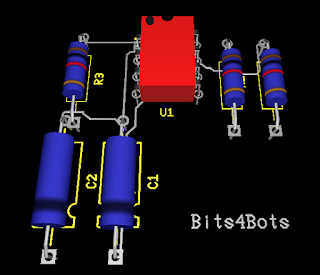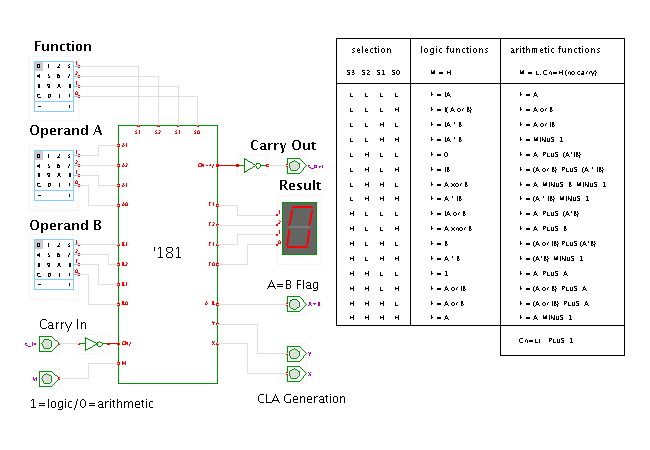## Tuesday, June 30, 2015

### HP 50g: Magnetism - Straight Wire & more

magnetic field is the magnetic effect of electric currents and magnetic materials

In this blog, using the HP 50g, I will show you how to get to the built in equation library and solve equations for
straight wire magnetism. The units for such is Tesla.
If you are required to answer in Gauss you will need to convert.
After turning on your HP 50g:
1. Press APPS
2. Scroll down to #12 or type in 12 (Equation Library)
3. Press OK. If Soft Menu mode is on you will see four boxes. Eqlib  Colib Mes Utils. Choose EQLIB. This is for the equation library.
4. In the library there are many choices. Today we will explore Magnetism. Go ahead and scroll down, press ENTER.
5. This brings us to today's topic. Straight Wire. Press ENTER. You should now see the see the equation. The soft key choices are now
• Solv Equ Vars Pic -->Stk Exit

1. PIC will show you a visual of the straight wire equation.
2. Vars gives a description of each variable that can be used in the equation.
3. EQN is the equation itself. The --> Stk puts the equation onto the stack.
4. The main soft key choice is Solv. This is the equation solver. To enter numerical information you must first key in the constant ex: 5_cm radius and 4_A current; -press 5 then press F2B the key directly under the r soft key menu, then 4 and F4Dfor current...soft key menu I.
5. Now to solve for B we must first press the White Right Shift key <-- and then F5E (B soft key menu)
Then solver should output the answer. Play around with different equations to get a feel for the powerful HP 50g!

Please subscribe for more great content.

## Wednesday, June 17, 2015

### 555 Timer: Ultiboard Design by Bits

The RED 555 Timer.This is a simple 555 timer circuit however, it has been redesigned by Bits. My idea of circuits and TTL components should have a bit more color options and since they (colorful TTL's) don't exactly exist I thought I'd just give the world a taste of what future circuits could look like when future engineers take office!
I used Multisim software and Ultiboard to come up with this unique red color for the 555 Timer. Ultiboard allows the user to modify almost any component on the circuit. Another fantastic feature was the option to change the shape of the pcb board. No more boring rectangles! I have included some of the different shapes that were possible. Here's a quick video!

## Tuesday, June 16, 2015

### NI Multisim: 74ls181 ALU 4 Bit Arithmetic Logic Unit

Written by Larsha Johnson
September 6/16/2015

74ls181 ALU 4 Bit Arithmetic Logic Unit# 74ls181 ALU 4-Bit Logic Unit TTL

What does a 4-Bit ALU do?

Written by: Larsha Johnson
6/16/2015

The 74181 is still used today in retro hacker projects. Here's ow it works and why it's so strange. Is it an all in one logic chip? Well, yes. Since it provides 16 Arithmetic Operations Add, Subtract, Compare, Double, Plus Twelve Other Arithmetic Operations as well as provides all 16 Logic Operations of Two Variables Exclusive — OR( XOR), Compare, AND, NAND, OR, NOR, Plus Ten other Logic Operations!

Why was it designed? To make computing faster in comparison with standard logic gates that was what available at the time. In March 1970, Texas Instruments introduced the 74181 Arithmetic / Logic Unit (ALU) chip, which put a full 4-bit ALU on one fast TTL chip. This chip provided 32 arithmetic and logic functions, as well as carry lookahead for high performance.

The SN54/74LS181 is a 4-bit Arithmetic Logic Unit (ALU) which can perform all the possible 16 logic, operations on two variables and a variety of arithmetic operations.

The 74181 ALU (arithmetic/logic unit) chip powered many of the minicomputers of the 1970s: it provided fast 4-bit arithmetic and logic functions, and could be combined to handle larger words, making it a key part of many CPUs.

The 74181 is a 4-bit slice arithmetic logic unit (ALU), implemented as a 7400 series TTL integrated circuit. The first complete ALU on a single chip, it was used as the arithmetic/logic core in the CPUs of many historically significant minicomputers and other devices.

The 74181 represents an evolutionary step between the CPUs of the 1960s, which were constructed using discrete logic gates, and today's single-chip microprocessor CPUs. Although no longer used in commercial products, the 74181 is still referenced in computer organization textbooks and technical papers. It is also sometimes used in 'hands-on' college courses, to train future computer architects.

• Provides 16 Arithmetic Operations Add, Subtract, Compare, Double,
Plus Twelve Other Arithmetic Operations
Provides all 16 Logic Operations of Two Variables Exclusive — OR,
Compare, AND, NAND, OR, NOR, Plus Ten other Logic Operations
• Full Lookahead for High Speed Arithmetic Operation on Long Words
• Input Clamp Diodes

FUNCTIONAL DESCRIPTION

The SN54/74LS181 is a 4-bit high speed parallel Arithmetic Logic Unit (ALU). Controlled by the four Function Select Inputs (S0 . . . S3) and the Mode Control Input (M), it can perform all the 16 possible logic operations or 16 different arithmetic operations on active HIGH or active LOW operands. The Function Table lists these operations.

The combinational logic circuitry of the 74181 integrated circuit

Reference articles### Traffic Light in NI Multisim using Programmable Logic Devices (PLD)

Traffic Light in Multisim using PLC Written by Larsha Johnson 9/27/2021 Programmable Logic Devices (PLDs) are integrated circuits that conta...